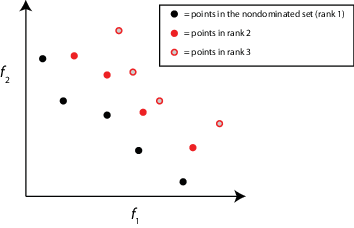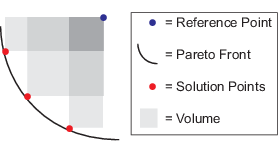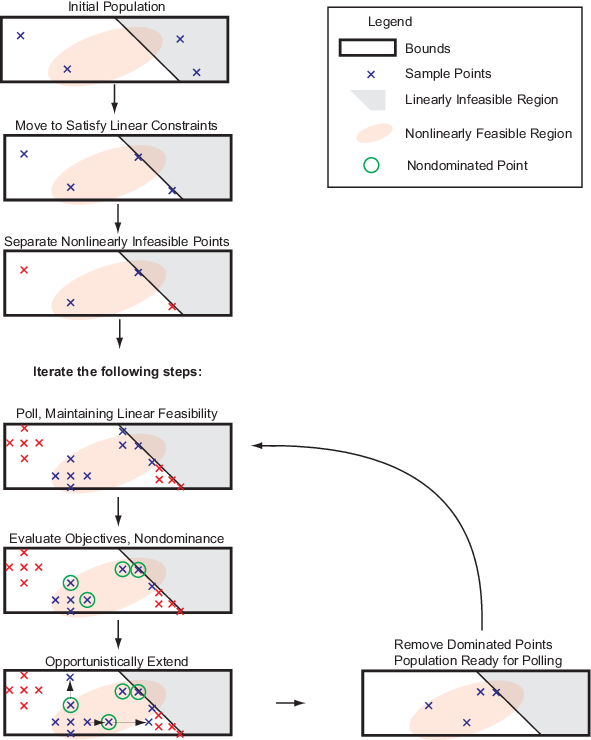## `paretosearch` Algorithm

### `paretosearch` Algorithm Overview

The `paretosearch` algorithm uses pattern search on a set of points to search iteratively for nondominated points. See Multiobjective Terminology. The pattern search satisfies all bounds and linear constraints at each iteration.

Theoretically, the algorithm converges to points near the true Pareto front. For a discussion and proof of convergence, see Custòdio et al. , whose proof applies to problems with Lipschitz continuous objectives and constraints.

### Definitions for `paretosearch` Algorithm

`paretosearch` uses a number of intermediate quantities and tolerances in its algorithm.

QuantityDefinition
Rank

The rank of a point has an iterative definition.

• Nondominated points have rank 1.

• For any integer `k` > 1, a point has rank `k` when the only points that dominate it have rank strictly less than `k`.Volume

Hypervolume of the set of points p in objective function space that satisfy the inequality, for every index j,

fi(j) < pi < Mi,

where fi(j) is the ith component of the jth objective function value in the Pareto set, and Mi is an upper bound for the ith component for all points in the Pareto set. In this figure, M is called the Reference Point. The shades of gray in the figure denote portions of the volume that some calculation algorithms use as part of an inclusion-exclusion calculation.For details, see Fleischer .

`paretosearch` calculates the volume only when the number of nondominated points exceeds the number of objectives. `paretosearch` uses the reference point M = `max(pts,[],1) + 1`. Here, `pts` is a matrix whose rows are the points.

Volume change is one factor in stopping the algorithm. For details, see Stopping Conditions.

Distance

Distance is a measure of the closeness of an individual to its nearest neighbors. The `paretosearch` algorithm measures distance among individuals of the same rank. The algorithm measures distance in objective function space.

The algorithm sets the distance of individuals at the extreme positions to `Inf`. For the remaining individuals, the algorithm calculates distance as a sum over the dimensions of the normalized absolute distances between the individual's sorted neighbors. In other words, for dimension `m` and sorted, scaled individual `i`:

```distance(i) = sum_m(x(m,i+1) - x(m,i-1))```.

The algorithm sorts each dimension separately, so the term neighbors means neighbors in each dimension.

Individuals of the same rank with a higher distance have a higher chance of selection (higher distance is better).

Distance is one factor in the calculation of the spread, which is part of a stopping criterion. For details, see Stopping Conditions.

Spread is a measure of the movement of the Pareto set. To calculate the spread, the `paretosearch` algorithm first evaluates σ, the standard deviation of the crowding distance measure of points on the Pareto front with finite distance. Q is the number of these points, and d is the average distance measure among these points. The algorithm then evaluates μ, the sum over the `k` objective function indices of the norm of the difference between the current minimum-value Pareto point for that index and the minimum point for that index in the previous iteration. The spread is then

`spread` = (μ + σ)/(μ + Qd).

The spread is small when the extreme objective function values do not change much between iterations (that is, μ is small) and when the points on the Pareto front are spread evenly (that is, σ is small).

`paretosearch` uses the spread in a stopping condition. Iterations halt when the spread does not change much. For details, see Stopping Conditions.

`ParetoSetChangeTolerance`Stopping condition for the search. `paretosearch` stops when the volume, spread, or distance does not change by more than `ParetoSetChangeTolerance` over a window of iterations. For details, see Stopping Conditions.
`MinPollFraction`

Minimum fraction of locations to poll during an iteration. `paretosearch` polls at least `MinPollFraction`*(number of points in pattern) locations. If the number of polled points gives a nondominated point, the poll is considered a success. Otherwise, `paretosearch` continues to poll until it either finds a nondominated point or runs out of points in the pattern.

This option does not apply when the `UseVectorized` option is `true`. In that case, `paretosearch` polls all pattern points.

### Sketch of `paretosearch` Algorithm### Initialize Search

To create the initial set of points, `paretosearch` generates `options.ParetoSetSize` points from a quasirandom sample based on the problem bounds, by default. For details, see Bratley and Fox . When the problem has over 500 dimensions, `paretosearch` uses Latin hypercube sampling to generate the initial points.

If a component has no bounds, `paretosearch` uses an artificial lower bound of `-10` and an artificial upper bound of `10`.

If a component has only one bound, `paretosearch` uses that bound as an endpoint of an interval of width `20 + 2*abs(bound)`. For example, if there is no upper bound for a component and there is a lower bound of 15, `paretosearch` uses an interval width of 20 + 2*15 = 55, so uses an artificial upper bound of 15 + 55 = 70.

If you pass some initial points in `options.InitialPoints`, then `paretosearch` uses those points as the initial points. `paretosearch` generates more points, if necessary, to obtain at least `options.ParetoSetSize` initial points.

`paretosearch` then checks the initial points to ensure that they are feasible with respect to the bounds and linear constraints. If necessary, `paretosearch` projects the initial points onto the linear subspace of linearly feasible points by solving a linear programming problem. This process can cause some points to coincide, in which case `paretosearch` removes any duplicate points. `paretosearch` does not alter initial points for artificial bounds, only for specified bounds and linear constraints.

After moving the points to satisfy linear constraints, if necessary, `paretosearch` checks whether the points satisfy the nonlinear constraints. `paretosearch` gives a penalty value of `Inf` to any point that does not satisfy all nonlinear constraints. Then `paretosearch` calculates any missing objective function values of the remaining feasible points.

### Note

Currently, `paretosearch` does not support nonlinear equality constraints `ceq(x) = 0`.

### Create Archive and Incumbents

`paretosearch` maintains two sets of points:

• `archive` — A structure that contains nondominated points associated with a mesh size below `options.MeshTolerance` and satisfying all constraints to within `options.ConstraintTolerance`. The `archive` structure contains no more than `2*options.ParetoSetSize` points and is initially empty. Each point in `archive` contains an associated mesh size, which is the mesh size at which the point was generated.

• `iterates` — A structure containing nondominated points and possibly some dominated points associated with larger mesh sizes or infeasibility. Each point in `iterates` contains an associated mesh size. `iterates` contains no more than `options.ParetoSetSize` points.

### Poll to Find Better Points

`paretosearch` polls points from `iterates`, with the polled points inheriting the associated mesh size from the point in `iterates`. The `paretosearch` algorithm uses a poll that maintains feasibility with respect to bounds and all linear constraints.

If the problem has nonlinear constraints, `paretosearch` computes the feasibility of each poll point. `paretosearch` keeps the score of infeasible points separately from the score of feasible points. The score of a feasible point is the vector of objective function values of that point. The score of an infeasible point is the sum of the nonlinear infeasibilities.

`paretosearch` polls at least `MinPollFraction`*(number of points in pattern) locations for each point in `iterates`. If the polled points give at least one nondominated point with respect to the incumbent (original) point, the poll is considered a success. Otherwise, `paretosearch` continues to poll until it either finds a nondominated point or runs out of points in the pattern. If `paretosearch` runs out of points and does not produce a nondominated point, `paretosearch` declares the poll unsuccessful and halves the mesh size.

If the poll finds nondominated points, `paretosearch` extends the poll in the successful directions repeatedly, doubling the mesh size each time, until the extension produces a dominated point. During this extension, if the mesh size exceeds `options.MaxMeshSize` (default value: `Inf`), the poll stops. If the objective function values decrease to `-Inf`, `paretosearch` declares the problem unbounded and stops.

### Update `archive` and `iterates` Structures

After polling all the points in `iterates`, the algorithm examines the new points together with the points in the `iterates` and `archive` structures. `paretosearch` computes the rank, or Pareto front number, of each point and then does the following.

• Mark for removal all points that do not have rank 1 in `archive`.

• Mark new rank `1` points for insertion into `iterates`.

• Mark feasible points in `iterates` whose associated mesh size is less than `options.MeshTolerance` for transfer to `archive`.

• Mark dominated points in `iterates` for removal only if they prevent new nondominated points from being added to `iterates`.

`paretosearch` then computes the volume and distance measures for each point. If `archive` will overflow as a result of marked points being included, then the points with the largest volume occupy `archive`, and the others leave. Similarly, the new points marked for addition to `iterates` enter `iterates` in order of their volumes.

If `iterates` is full and has no dominated points, then `paretosearch` adds no points to `iterates` and declares the iteration to be unsuccessful. `paretosearch` multiplies the mesh sizes in `iterates` by 1/2.

### Stopping Conditions

For three or fewer objective functions, `paretosearch` uses volume and spread as stopping measures. For four or more objectives, `paretosearch` uses distance and spread as stopping measures. In the remainder of this discussion, the two measures that `paretosearch` uses are denoted the applicable measures.

The algorithm maintains vectors of the last eight values of the applicable measures. After eight iterations, the algorithm checks the values of the two applicable measures at the beginning of each iteration, where ```tol = options.ParetoSetChangeTolerance```:

• ```spreadConverged = abs(spread(end - 1) - spread(end)) <= tol*max(1,spread(end - 1));```

• ```volumeConverged = abs(volume(end - 1) - volume(end)) <= tol*max(1,volume(end - 1));```

• ```distanceConverged = abs(distance(end - 1) - distance(end)) <= tol*max(1,distance(end - 1));```

If either applicable test is `true`, the algorithm stops. Otherwise, the algorithm computes the max of squared terms of the Fourier transforms of the applicable measures minus the first term. The algorithm then compares the maxima to their deleted terms (the DC components of the transforms). If either deleted term is larger than `100*tol*(max of all other terms)`, then the algorithm stops. This test essentially determines that the sequence of measures is not fluctuating, and therefore has converged.

Additionally, a plot function or output function can stop the algorithm, or the algorithm can stop because it exceeds a time limit or function evaluation limit.

### Returned Values

The algorithm returns the points on the Pareto front as follows.

• `paretosearch` combines the points in `archive` and `iterates` into one set.

• When there are three or fewer objective functions, `paretosearch` returns the points from the largest volume to the smallest, up to at most `ParetoSetSize` points.

• When there are four or more objective functions, `paretosearch` returns the points from the largest distance to the smallest, up to at most `ParetoSetSize` points.

### Modifications for Parallel Computation and Vectorized Function Evaluation

When `paretosearch` computes objective function values in parallel or in a vectorized fashion (`UseParallel` is `true` or `UseVectorized` is `true`), there are some changes to the algorithm.

• When `UseVectorized` is `true`, `paretosearch` ignores the `MinPollFraction` option and evaluates all poll points in the pattern.

• When computing in parallel, `paretosearch` sequentially examines each point in `iterates` and performs a parallel poll from each point. After returning `MinPollFraction` fraction of the poll points, `paretosearch` determines if any poll points dominate the base point. If so, the poll is deemed successful, and any other parallel evaluations halt. If not, polling continues until a dominating point appears or the poll is done.

• `paretosearch` performs objective function evaluations either on workers or in a vectorized fashion, but not both. If you set both `UseParallel` and `UseVectorized` to `true`, `paretosearch` calculates objective function values in parallel on workers, but not in a vectorized fashion. In this case, `paretosearch` ignores the `MinPollFraction` option and evaluates all poll points in the pattern.

### Run `paretosearch` Quickly

The fastest way to run `paretosearch` depends on several factors.

• If objective function evaluations are slow, then it is usually fastest to use parallel computing. The overhead in parallel computing can be substantial when objective function evaluations are fast, but when they are slow, it is usually best to use more computing power.

### Note

Parallel computing requires a Parallel Computing Toolbox™ license.

• If objective function evaluations are not very time consuming, then it is usually fastest to use vectorized evaluation. However, this is not always the case, because vectorized computations evaluate an entire pattern, whereas serial evaluations can take just a small fraction of a pattern. In high dimensions especially, this reduction in evaluations can cause serial evaluation to be faster for some problems.

• To use vectorized computing, your objective function must accept a matrix with an arbitrary number of rows. Each row represents one point to evaluate. The objective function must return a matrix of objective function values with the same number of rows as it accepts, with one column for each objective function. For a single-objective discussion, see Vectorize the Fitness Function (`ga`) or Vectorized Objective Function (`patternsearch`).

 Custòdio, A. L., J. F. A. Madeira, A. I. F. Vaz, and L. N. Vicente. Direct Multisearch for Multiobjective Optimization. SIAM J. Optim., 21(3), 2011, pp. 1109–1140. Preprint available at https://estudogeral.sib.uc.pt/bitstream/10316/13698/1/Direct%20multisearch%20for%20multiobjective%20optimization.pdf.

 Bratley, P., and B. L. Fox. Algorithm 659: Implementing Sobol’s quasirandom sequence generator. ACM Trans. Math. Software 14, 1988, pp. 88–100.

 Fleischer, M. The Measure of Pareto Optima: Applications to Multi-Objective Metaheuristics. In "Proceedings of the Second International Conference on Evolutionary Multi-Criterion Optimization—EMO" April 2003 in Faro, Portugal. Published by Springer-Verlag in the Lecture Notes in Computer Science series, Vol. 2632, pp. 519–533. Preprint available at http://www.dtic.mil/get-tr-doc/pdf?AD=ADA441037.

Watch now